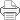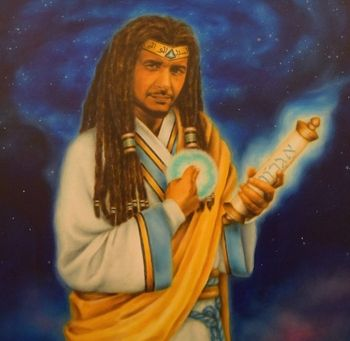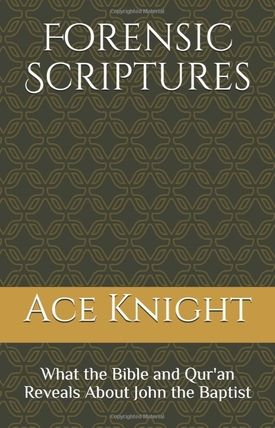Tuesday September 17, 2019
SNc Channels:

Search

Jun-27-2019 01:30# Forensic Scriptures: Manifesto 13

The Key and The Sublime Qur'an
Section 5John the Baptist by Toni L. Taylor

(BOSTON) - "If all of Ace Knight’s research and the conclusions he draws from it prove to be valid, then the traditional view of John the Baptist/Yahya, both scholarly and conventional, Jewish, Christian and Muslim, will be subjected to a tidal wave of revision and reconsideration.
"This will also affect most extant translations of the Quran into English, with the exception of The Sublime Quran by Dr. Laleh Bakhtiar which incorporates all of his results that relate to Quranic verses.
"Additionally, the great collections of medieval Islamic commentaries, both Sunni and Shia, which often repeat such colorful Biblical stories as the beheading of John the Baptist, will have to be viewed more critically. Such revisionism is sure to meet with a strong opposition." -Award winning journalist Tim King

"Ace Knight is one of the leading authorities, if not the leading authority, on the research of John the Baptist.
His latest explorations in the field of scriptural numerology are producing amazing and thought-provoking results." - Shaykh

Muhammad Nur Abdus Salam: www.islamicbookstore.com/b7555.html

World-renowned scholar Dr. Mahmoud Ayoub said, “I met Ace Knight at an unusual interfaith conference in New York City. He was a professional, but also a passionate man. I soon discovered that my friend is at once a writer, an singer, and a man of many hobbies. His book on who actually was on the cross caused a stir in academia, yet Ace is not an academician.
"I was surprised to hear my friend’s songs, which again were artistically moving; yet, my friend is neither a professional musician nor a poet. Ace and I discovered a spiritual affinity between us, which I cannot explain. It is beautiful and enriching.”

"Or do they have gods who can protect them against us?" (Quran 21:43)
تَمْنَعُهُم مِّن دُونِنَا
Total Gematrical value for each word:
4–4–5—7–5–4= 29
5—4= 9
4—6—5—5= 20
Total— 2+9+9+2+0= 22

Al-Mani` is one of God's Attributes and is derived from man`, the opposite of giving or granting.
It also means: to protect, to stop one thing from harming another or one group of people from annihilating another. Qul

“Repent for the Kingdom of Heaven is at Hand” Matthew 3:2—3+2= 5 —Yahya-Yuhanan aka John the Baptist

The Code 19 was discovered in 1974 exactly 1406 lunar years since the revelation of the Quran

Discovery—1406—1974 1+4+0+6+1+9+7+4= 32—3+2= 5

1406 is a multiple of 2x19x37 2+1+9+3+7= 22

14 different sets +14 centuries= 28 (Y10H8Y10—Yahya—He lives)— 2+8= 10Y

Al’fatiha The Opening

[1:1] In the name of GOD, Most Gracious, Most Merciful

The Key 19/22 Letters

بِسْمِ اللَّهِ الرَّحْمَٰنِ الرَّحِيمِ

Appears as 19 Letters

B—S—M—A—L—L—H—A—L—R—H—M—N—A—L—R—H—Y—M—19

Floating alif and double Ra added

B—S—M—A—L—L—H—A—L—R—R—H—M—N—A—L—R—R—H—Y—M= 22

19:22—19+22= 41—4+1= 5

B—S—M—A—L—L—H—A—L—R—H—M—N—A—L—R—H—Y—M

2—60—40—1—30—30—5—1—30—200—8—4—50—1—30—200—8—10—40= 786

786—7+8+6= 21—2+1= 3

Add the letter alif that is floating over the mim in Rahman. That mim is counted as a 5, and for good reason:

Mim 40+Alif 1= 41= 5

Rahman (Gracious). The number 5 symbolizes God’s Grace

الرَّحْمَٰنِ

A—L—R—H—M—N= 5

A1—L30—R200—H8—M40—N50= 329—3+2+9= 14—1+4= 5 (Qur’an confirms)

2—60—40—1—30—30—5—1—30—200—8—5—50—1—30—200—8—10—40= 787

By adding the number of God’s grace: 787—7+8+7= 22

بِسْمِ اللَّهِ

Ism: Name—Allah: The God

ISM from the root letters S—M—W— 6–4–6—16—1+6= 7

ISM appears 22 times in the sacred text.

A—L—L—H= 1–3–3–5= 12—1+2= 3

7+3=10Y

A—L—L—H —English Letters

Numerical Value: 1—12—12—8= 15YH

Rahman (Grace) The number 5 symbolizes God’s Grace بِسْمِ اللَّهِ الرَّحْمَٰنِ

In the Name of God—The Gracious (Rahman):

Floating alif over mim added:

B—S—M—A—L—L—H—A—L—R—H—M—A—N= 14—1+4= 5

رَّحْمَٰن

Rahman—Double Ra—2+2+8+4+1+5= 22

Raheem—Double Ra— 2+2+8+1+4=17—1+7= 6

22+6= 28

28 Letters in the Arabic Alphabet

28 is the gematrical value of the Name Yahya—(He Lives)

A—L—R—H—M—N= 1+3+2+8+4+5= 23— 5

A—L—R—H—Y—M= 1+ 3+ 2+ 8+ 1+ 4= 19

19/5— Q. 19:5 Protector (Waliy/Yahya)

19:5—1+9+5= 15YH

[1:2] Praise be to GOD, Lord of the universe.

[1:3] The Gracious, The Merciful.

A—L—R—H—M—N= 1+3+2+8+4+5= 23— 5

A—L—R—H—Y—M= 1+ 3+ 2+ 8+ 1+ 4= 19

[1:4] Master of the Day of Judgment.

1+4= 5

[1:5] You alone we worship; You alone we ask for help.

1:5— 15YH

[1:6–7] Guide us in the right path; the path of those whom You blessed; not of those who have deserved wrath, nor of the strayers.

1:6–7—1+6+7= 14—1—4= 5

1:6–1:7–1+6+1+7= 15YH—15 + 7 Signs= 22

The Qur’an

Quran 114 Chapters— 6,236 Verses

1+1+4+6+2+3+6=23—2+3= 5

AND, INDEED, We have bestowed upon thee seven (7) of the oft repeated [verses/signs], and [have, thus, laid open before thee this sublime Qur'an 15:87

Chapter 1—The Opener: Al Fatiha—The Key 7 Signs

From the Key: The Sublime Quran—113 Chapters 6229 Verses

1+1+3= 5 –6+2+2+9= 19—19/5

The 7 Signs + 19/5—1+9+5+7= 22SEE ALSO: globalnewscentre.com

Chapter 15:87—1+5+8+7= 21+ 7 Signs= 28 (YHY)—10Y

Strain not thine eyes at what We have bestowed on certain classes of them, nor grieve over them: but lower thy wing to the believers. 15:88—1+5+8+8= 22

“The Key” and 5 moves to Victory

From The Key to the last chapter revealed by God:

On every 22nd Chapter:

22—44—66—88—110

Chapter and verses:

1+1+0+1+1+1+0+2+1+1+0+3= 12— 1+2= 3 Perfect order

The Key 7 Verses—The Victory 3 Verses

7+3= 10Y

“A word” linked to Jesus occurs 2X in the sacred text: that's "22"

O Mary! Behold, God gives you good news of a word from Him,

who shall become known as the Messiah, Jesus, son of Mary..."

(Quran 3:45)

"The Messiah, Jesus son of Mary, was only a messenger of God, and His word which He conveyed unto Mary..."

(Quran 4:171)

3+4+5+4+1+7+1= 25—also, mentioned 25X in the Qur’an 25X25= 50— 5

Again, from The Key to The Victory:

22—44—66—81—110

110:1—3

Chapter and verses:

1+1+0+1+1+1+0+2+1+1+0+3= 12— 1+2= 3 Perfect order

Add code 22— 22+3= 25 — 2+5= 7

A word (25) from God (7)

25+7= 32—3+2= 5

5 Occurrences of 22:

22—44—66—88—110

2+2+4+4+6+6+8+8+1+1+0= 42

4+2= 6 —Add code 22—22+6= 28 (Yahya)

Add the 5 occurrences of 22— 28+5= 33

Code 22+33= 55= 10Y

19/5

10/5—15

Yahya confirms a word from God

God gives thee good tidings of Yahya—one who establishes the Word of God as true—

a chief and concealer of secrets and a Prophet among the ones in accord with morality.

Hasur—Concealer of Secrets—Root: HSR, gematrical value 19

3:39—3+3+9= 15YH

Yahya is also mentioned 5 times in the Quran

The names:

Zakariah—Mary—Jesus—John

Zakariyah—Maryam—Esa—Yahya

Gematrical Values:

Z—K—R= 11—M—R—Y—M=11

E—S—A= 5—Y—H—Y= 28

Zachariah—Mary— 11+11= 22

Jesus—John—5+28= 33 or, 10/5YH

Total for names: 1+1+1+5+2+8= 19

Yahya 28—2+8= 10—Esa 5

10/5YH

Esa strengthened with the Holy Spirit

Mentioned 25X in the Quran—2+5= 7

He said: So (it will be). Thy Lord saith: It is easy for Me. And (it will be) that We may make of him a revelation

for mankind and a mercy from Us, and it is a thing ordained.

19:21—1+9+2+1= 13

15 Arabic root words in this verse, and 43 root letters

Adding every root letter and it’s gematrical value we get 9

15 Arabic words—43 Root Letters—gematrical value of each root letter 9

1+5+4+3+9= 22

The 22nd root letter lands on the Ayn

which is the first letter in the name of Jesus in the Quran:

Jesus—Ayn—Ya—Seen—Gematrical value 70—10—60—140—1+4= 5

وَلِنَجْعَلَهُ —walinajʿalahu—and We will make him A Sign (revelation)

وَلِنَجْعَلَهُ

Gematrical value for the letters in this word:

6+3+5+0+3+7+0+3+5= 32—3+2= 5

A Sign for mankind and a mercy from us:

So she conceived him and she went apart with him to a farther place. Q. 19:22 (19/22)

19:22—1+9+2+2= 14“5”

19/5—Q. 19:5 Protector (Yahya mentioned 5X)

And (Jesus) shall be a Sign (for the coming of) the Hour (of Judgment):

therefore have no doubt about the (Hour), but follow ye Me: this is a Straight Way.

Q. 43:61—4+3+6+1= 14—1+4= 5

Jesus and Life—force (Min Rouhina) 21:91, 66:12

2+1+9+1+6+6+1+2= 28— (Yahya 28)

So we breathed into her of Our Spirit

فَنَفَخْنَا —Root letters ن ف خ

Gematrical Value: 5—8—6= 19

فِيهِ—Root letters. ف ى

Gematrical value: 8—1= 9

19+9= 28 (He Lives)

Chapter 1—The Opener: Al Fatiha—The Key 7 Signs

From the Key: The Sublime Quran—113 Chapters 6229 Verses

1+1+3= 5 –6+2+2+9= 19—19/5

The 7 Signs + 19/5—1+9+5+7= 22

The Arabic letter S appears in chapters 7—19—38

7+1+9+3+8= 28

Note: The letter S lands as the 19th letter in the English Alphabet

“By the Quran, Possessor of the Remembrance.”

وَالْقُرْءَانِ ذِي الذِّكْرِ

Gematrical value for root letters= 19

(This is) a Book which We have sent down to you, full of blessings that they may ponder over its Verses, and that men of understanding may remember.” [Saad, 38:29]

3+8+2+9= 22

Chapter 41: Detailed—5 -- 4+1= 5

Chapter 41, Surah Fuṣṣilat : Ḥāʾ Mīm

22nd in Sequential order (Muqatta’at letters)

41: 0—In the name of God, Most Gracious, Most Merciful—19 Letters

4+1= 5—19/5

41:1 H. M.= 12—1+2= 3

The first occurrence of the special letter H is in Chapter 20 Ta Ha

The first occurrence of the Special letter M is in Chapter 2 Baqara

20+2= 22

Using 19 Letters as Key+ H. M. Gematrical Value of 3—19+3= 22

19/22

4+1+1= 6+ 22= 28YHY

4+1+1+2+2= 10Y

41:2 A revelation from the Most Gracious, Most Merciful.

In the verse appears 19 letters, and adding the doublers we get a total of 22 Arabic letters 19/22

4+1+2= 7 (Signs)

الرَّحْمَنِ الرَّحِيمِ

A—L—R—H—M—N= 1+3+2+8+4+5= 23— 5

A—L—R—H—Y—M= 1+ 3+ 2+ 8+ 1+ 4= 19

19/5

ر ح م —ر ح م G..Value.—14+14= 28—(28 letters Arabic Alphabet)

(Q. 19:5 Protector—28 Gematrical Value for the name Yahya)

41:3 A scripture whose signs provide the complete details,

in an Arabic Quran, for people who know.

G.Value.—A book detailed—28Y

G.Value—Signs Quran—13

2+8+1+3= 14—1+4= 5

Arabic—11—For a people 11— 22

Who Know 14— 1+4= 5

*****(See Chapter 13:1—A.L.M.R. These are the signs of the Book

المر تِلْكَ آيَاتُ الْكِتَابِ — Gematrical Value for Root letters 10+9+3= 22)

The Children of Israel-The Night Travel

17:1 “The Children of Israel”

Glory be He who summoned His servant during the night,

from the forbidden station to the highest station

whose surroundings we have blessed, in order to show him some of our signs.

He is the Hearer, the Seer.

سُبْحَانَ الَّذِي أَسْرَىٰ بِعَبْدِهِ

“Glory be He who summoned His servant”

Total Root letters for these words:

س ب ح — ا ل ل ذ— ىس ر و —ع ب د

S6+B2+H8= 16

A1+L30+L30+DH7+Y10= 15

S6+R2+W6= 14

AYN7+B2+D4= 13

Total—1+6+1+5+1+4+1+3= 22

This verse is speaking about Zakariyah who was in the forbidden chamber with Maryam,

and then taken to a higher state of conscious.

Zakariyah and Maryam:

Z—K—R= 11—M —R—Y—M= 11

11+11= 22

بِعَبْدِهِ

His Servant

The words His servant is linked to only one

prophetic name in the whole of the Qur’an:

(His servant Zakariyah 19:2)

لَيْلًا مِّنَ الْمَسْجِدِ الْحَرَامِ

“By night, from the forbidden chamber”

30+10+30= 7

40+50= 9

6+3+4= 13

8+20+40= 14

Total: 7+9+1+3+1+4= 25

Jesus the son of Mary is mentioned 25x in the Quran

إِلَى الْمَسْجِدِ الْأَقْصَى الَّذِي بَارَكْنَا

...to the highest station

whose surroundings we have blessed

1+30+10= 5

6+3+4= 13

100+90+6= 16

1+30+30+7+10= 24

2+200+20= 6

Total: 5+1+3+1+6+2+4+6= 28 (Gematrical value for Yahya)

“whose surroundings we have blessed”

حَوْلَهُ

This Arabic word ḥawlahu has a gematrical value of 22

إِنَّهُ هُوَ السَّمِيعُ الْبَصِيرُ

Q. 17:1: “...show him some of our signs. He is the Hearer, the Seer”

Root letters for these words:

1+10+1= 3

1—50—50= 11

5+6= 11

60+40+7= 17

2+90+20= 13

Total 3+1+1+1+1+1+7+1+3= 19

Chapter 19 “Maryam”

Q. 19:1—1+9+1= 11

كهيعص

5 Key words Maximum

19:2= 12 —1+2= 3

11+3= 14—1+4= 5

ذِكْرُ رَحْمَتِ رَبِّكَ عَبْدَهُ زَكَرِيَّا

A remembrance of the grace of thy Lord to His servant Zechariah

Total Gematrical Value for root letters= 19

19/5

ذِكْرُ —Remember—G.Value Root letters = 11

زَكَرِيَّا —Zakariayah— G. Value Root letters= 11

11+11= 22

فَنَادَتْهُ الْمَلَائِكَةُ وَهُوَ قَائِمٌ يُصَلِّي فِي الْمِحْرَابِ

“And the angels called to him as he stood praying in the high station” Q. 3:39

Q. 3:39—3+3+9= 15YH

فَنَادَتْهُ الْمَلَائِكَةُ —Fanādathu—Malāikatu

Gematrical values: 5+4+1= 10—4+3+2= 9

19

وَهُوَ قَائِمٌ

“as he stood in prayer”

Gematrical value for root letters: 22

19/22

الْمِحْرَابِ

Mihrab—High Station

This word has a gematrical value of 22 (See 17:1 word Aqsa High Station)

GOD gives you good news of Yahya

God—1+3+5= 9—Yahya—28

9+2+8= 19

Good—News 15YH

مُصَدِّقًا بِكَلِمَةٍ

Confirming— 9+4+100= 14—1+4= 5

a word—20—30—40= 9

9+5= 14—1+4= 5

(Jesus- Ayn70-Ya-10-Seen= 14-1+4= 5

وَسَيِّدًا وَحَصُورًا وَنَبِيًّا

“a chief and concealer of secrets and a Prophet”

Gematrical Value for these words (doublers added)

6+6+10+10+4= 18

6+8+9+6+2= 31

6+5+2+10+10= 15

Total—1+8+3+1+1+5= 19

Root letters for Hasur Concealer of Secrets: HSR= 19

Q. 17:1 His Servant Q. 19:2 His Servant Zakariyah

Q. 17:1 To the highest station Q. 3:39 Stood in the high station

Chapter 17:1 belongs to Zakariyah, not Muhammad.

"Or do they have gods who can protect them against us?." (Quran 21:43)

Al-Mani` is one of God's Attributes and is derived from man`, the opposite of giving or granting. It also means: to protect, to stop one thing from harming another or one group of people from annihilating another.

تَمْنَعُهُم مِّن دُونِنَا

Total Gematrical value for each word:

4–4–5—7–5–4= 29

5—4= 9

4—6—5—5= 20

Total— 2+9+9+2+0= 22

The number 15:

(A sacred number symbolic of the name of God, because the letters of the holy name YOD, HE, JAH, are equal, in the Hebrew mode of numeration by the letters of the alphabet, to fifteen; YOD is equal to ten, and HE is equal to five. Hence, from veneration for this sacred name, the Hebrews do not, in ordinary computations, when they wish to express the number fifteen, make use of these two letters, but of two others, which are equivalent to nine and six.) —F.M.D.

Normally we do not use the yod-hey - יה (Kah) to express the number fifteen (10 + 5) as it is one of the names of HaShem.

Thus, the world was created with the concept of fifteen. —Rabbi Dr. Hillel ben David (Greg Killian)

“It is He Who made the sun an illumination and the moon as a light and ordained its mansions so that you would know the number of the years and the reckoning. God created that only in Truth. He explains distinctly the signs for a folk who know.” Q. 10:5

_________________________________________

### Read full research here:

Contact Ace Knight: HijackedMediaDesigns@gmail.com

_________________________________________Name:

All comments and messages are approved by people and self promotional links or unacceptable comments are denied.

Jahta Dreadluv June 30, 2019 2:03 pm (Pacific time)

Blessed and big up Ace Knight and keep it coming...

©2019 Salem-News.com. All opinions expressed in this article are those of the author and do not necessarily reflect those of Salem-News.com.

Articles for June 26, 2019 | Articles for June 27, 2019 | Articles for June 28, 2019Support
Salem-News.com: